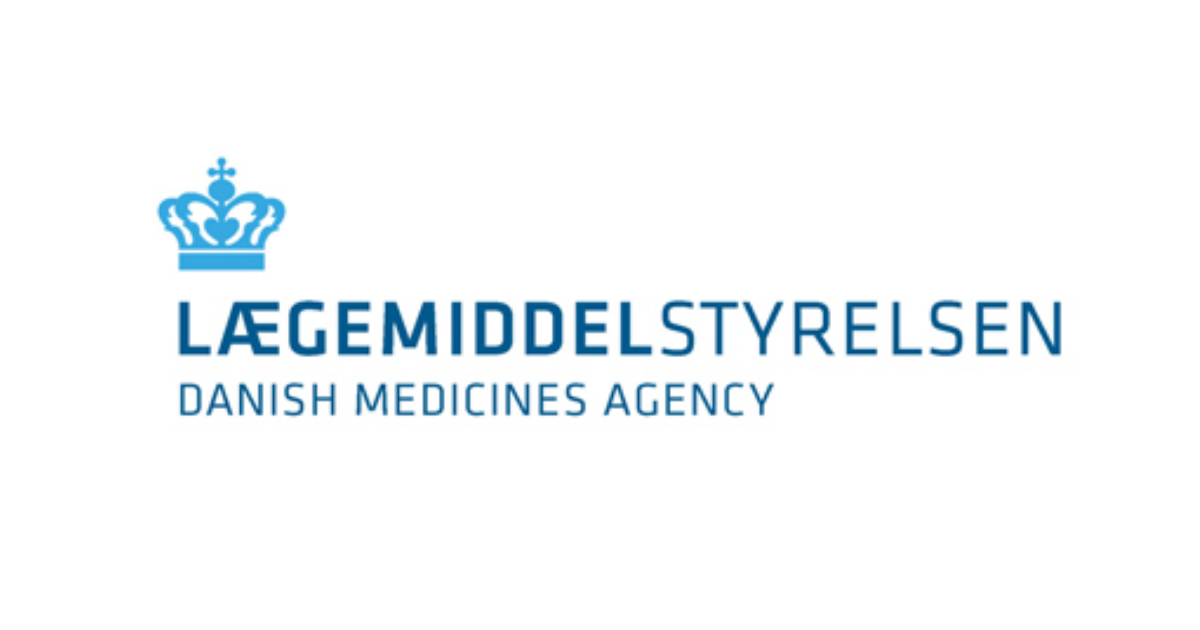# Conversion from pharmacy purchase price (PPP) to consumer price (ESP)

Updated 10 July 2023

The formula for calculation of the pharmacy profit margin was changed on 10 July 2023.

The constants of the formula are changed when it becomes necessary to adjust pharmacy earnings. The profit margin formula is laid down in the executive order on the calculation of consumer prices of medicinal products, etc.

Changes in the calculation of pharmacy profit margin generally take effect from the beginning of a new price period.

The following applies:

• Dispensing fee excluding VAT: DKK 8.00.
• Dispensing fee including VAT: DKK 10.00.

## PPP conversion formula

The conversion formula from PPP (pharmacy purchase price) to ESP (total consumer price) is as follows:

• ESP = 10,00 + 1,25 × (AIP × 0,068 + AIP + 5,46)

where the constant 10.00 is the dispensing fee including VAT.

Note that ESP is always rounded to the nearest amount divisible by DKK 0.05

## ESP conversion formula

The conversion formula from ESP to PPP is as follows:

• PPP = (0,8 x ESP - (8,00 + 5,46)) / (1 + 0,068)

A back calculation from PPP to ESP should always be performed to make sure that the figure has been rounded to the nearest amount divisible by DKK 0.05.

These calculations do not take into consideration any finishing fees.

The pharmacy profit margin is laid down in the Danish executive order no. 1022 of 28 June 2023 on calculation of consumer prices etc. of medicinal products.